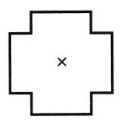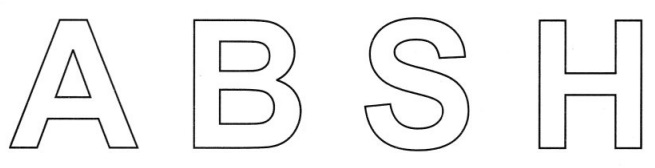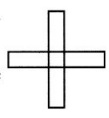### Grade 7 Maths Symmetry Multiple Choice Questions (MCQs)

1. The mirror image of ‘W’, when the mirror is placed vertically:
(a) V
(b) M
(c) S
(d) W

2. How many lines of symmetries are there in an equilateral triangle?
(a) 1
(b) 2
(c) 3
(d) 4

3. How many lines of symmetries are there in a rhombus?
(a) 1
(b) 2
(c) 3
(d) 4

4. How many lines of symmetries are there in regular pentagon?
(a) 3
(b) 2
(c) 5
(d) 4

5. How many lines of symmetries are there in rectangle?
(a) 1
(b) 2
(c) 3
(d) 4

6. Find the number of lines of symmetry of the following figure:
(a) 1
(b) 2
(c) 3
(d) 47. Find the number of lines of symmetry of the following figure:
(a) 1
(b) 2
(c) 3
(d) 48. Find the number of lines of symmetry in regular hexagon.
(a) 2
(b) 4
(c) 6
(d) 5

9. Letter ‘E’ of the English alphabet have reflectional symmetry (i.e., symmetry related to mirror reflection) about.
(a) a vertical mirror
(b) a horizontal mirror
(c) both (a) and (b)
(d) none of these

10. Letter ‘G’ of the English alphabet have reflectional symmetry (i.e., symmetry related to mirror reflection) about.
(a) a vertical mirror
(b) a horizontal mirror
(c) both (a) and (b)
(d) none of these

11. Letter ‘I’ of the English alphabet have reflectional symmetry (i.e., symmetry related to mirror refection) about.
(a) a vertical mirror
(b) a horizontal mirror
(c) both (a) and (b)
(d) none of these

12. Letter ‘T’ of the English alphabet have reflectional symmetry (i.e., symmetry related to mirror refection) about.
(a) a vertical mirror
(b) a horizontal mirror
(c) both (a) and (b)
(d) none of these

13. Find the number of lines of symmetry in a circle.
(a) 1
(b) 2
(c) 3
(d) none of these

14. Which of the followings has no line of symmetry:
(a) S
(b) A
(c) U
(d) H

15. Which letter look the same after reflection when the mirror is placed vertically.
(a) S
(b) P
(c) Q
(d) H

16. The order of the rotational symmetry of the parallelogram about the centre is:
(a) 0
(b) 1
(c) 2
(d) 3

17. The order of the rotational symmetry of the below left figure about the point marked ‘x’
(a) 0
(b) 1
(c) 2
(d) 318. The order of the rotational symmetry of the above sided right figure about the point marked ‘x’
(a) 0
(b) 1
(c) 2
(d) 3

19. The order of the rotational symmetry of the below left figure about the point marked
(a) 0
(b) 1
(c) 2
(d) 320. The order of the rotational symmetry of the above sided right figure about the point marked ‘x’
(a) o
(b) 1
(c) 2
(d) 3

### Grade 7 Maths Symmetry Fill In The Blanks

1. Rotation turns an object above a fixed point. This fixed point is called …………… .
2. Order of rotational symmetry of a circle is …………… .
3. Rhombus is a figure that has ……………. lines of symmetry and has a rotational symmetry of order …………….. .
4. ……………….. is a figure that has neither a line of symmetry non a rotational symmetry.
5. There is/are ………………… lines of symmetries in an isosceles triangle.

### Grade 7 Maths Symmetry True(T) Or False(F)

1. A regular pentagon has no lines of symmetry.
2. A scalene triangle has no line of symmetry.
3. Mirror reflection always leads to symmetry.
4. The number of lines of symmetry of a regular polygon is equal to the vertices of the polygon.
5. An angle has two line symmetry.

1. Draw all the lines of symmetry for the following letters of they exist.2. Tell whether each figure has rotational symmetry or not.1. Angle of rotation of a figure is 40°. What is the number of lines of symmetry.
2. What is the centre of rotation of a an equilateral triangle?
3. In the given figure:
(a) Find the order of rotational symmetry.
(b) Find the degree measure of the angle of rotation.4. Draw all lines of symmetry for each of the following figures given below: